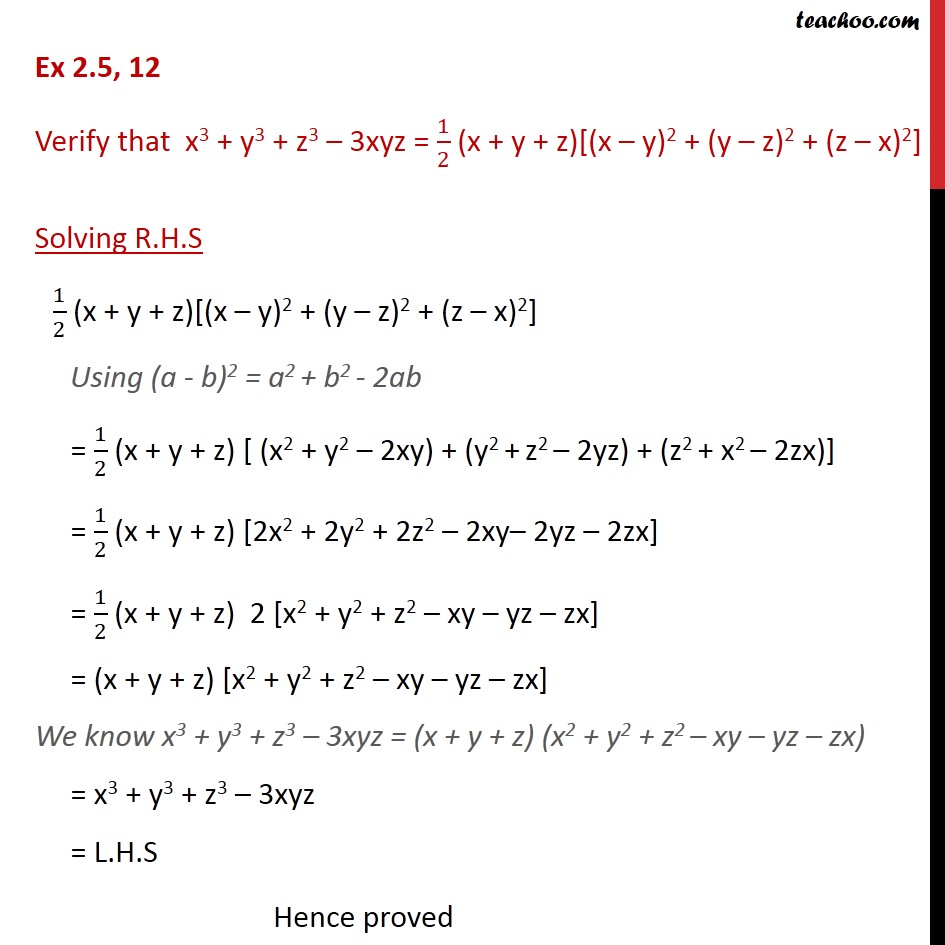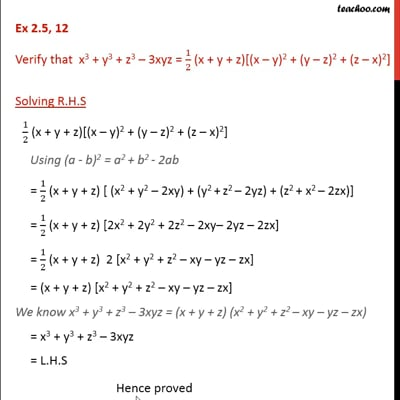Identity VIII

Chapter 2 Class 9 Polynomials (Term 2)
Concept wiseThis video is only available for Teachoo black users

### Transcript

Ex 2.5, 12 Verify that x3 + y3 + z3 – 3xyz = 1/2 (x + y + z)[(x – y)2 + (y – z)2 + (z – x)2] Solving R.H.S 1/2 (x + y + z)[(x – y)2 + (y – z)2 + (z – x)2] Using (a - b)2 = a2 + b2 - 2ab = 1/2 (x + y + z) [ (x2 + y2 – 2xy) + (y2 + z2 – 2yz) + (z2 + x2 – 2zx)] = 1/2 (x + y + z) [2x2 + 2y2 + 2z2 – 2xy– 2yz – 2zx] = 1/2 (x + y + z) 2 [x2 + y2 + z2 – xy – yz – zx] = (x + y + z) [x2 + y2 + z2 – xy – yz – zx] We know x3 + y3 + z3 – 3xyz = (x + y + z) (x2 + y2 + z2 – xy – yz – zx) = x3 + y3 + z3 – 3xyz = L.H.S